# The drag of an airfoil at zero angle of attack is a function of density, viscosity, and velocity, in addition to a length parameter

Download 2.42 Mb.
 Page 1/31 Date 20.05.2018 Size 2.42 Mb.
7.31 The drag of an airfoil at zero angle of attack is a function of density, viscosity, and velocity, in addition to a length parameter. A 1/10th scale model of an airfoil was tested in a wind tunnel at a Reynolds number of 5.5 x 106 , based on chord length. Test conditions in the wind tunnel air stream were 15 C and 10 atm absolute pressure. The prototype airfoil has a chord length of 2 m, and it is to be flown in air at standard conditions. Determine the speed at which the wind tunnel model was tested, and the corresponding prototype speed.
Rem = 5.5 x 106

Tm = 15o C = Tp  T = 1

pm = 10 atm, pp = 1 atm  p = 10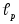= 2 m ; L = 1/10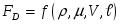Choose MLT as fundamental units
FD   V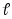MLT-2 ML-3 ML-1T-1 LT-1 L

Dimensional matrix

FD ,  ,  produce a 3 x 3 matrix with a non-zero determinant  Rank = 3

 L T M FD 1 -2 1  -3 0 1  -1 -1 1 V 1 -1 01 0 0

choose  , V ,as repeaters
Check on independence of the dimensions of the repeaters
L T M

 -3 0 1
V 1 -1 0  0  dimensions of  , V ,are independent1 0 0

Dimensionless groups: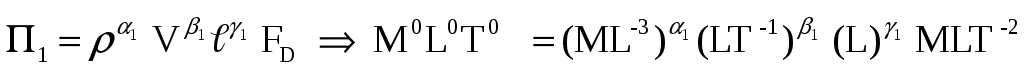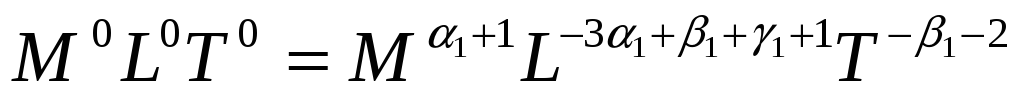 1 + 1 = 0  1 = -1 ;

-1 - 2 = 0  1 = -2; -3(-1) + (-2) + 1 + 1 = 0  1 = -2

Download 2.42 Mb.

Share with your friends:

The database is protected by copyright ©ininet.org 2020
send message